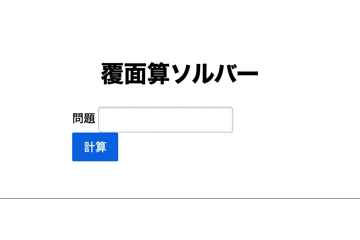## Introduction

Hello. It is 0kayu806059. This article is Qiita's first post. As the title says, this time I made a verbal arithmetic solver. It's a very simple web app, but I'd like to keep track of how it was completed.

## What is Verbal Arithmetic in the first place?

Verbal arithmetic is a puzzle in which you are given a formula in which some (or all) of the numbers are replaced with alphabets, so you can find the numbers that apply to each alphabet and make the formula hold. For example ABC + BAC = CACA Is given the formula. In this case, it's like thinking about what numbers A, B, and C will contain. It's the one you see when you take the junior high school exam. By the way, the answer is A = 2, B = 9, C = 1. When I actually substitute the number, We get 291 + 921 = 1212, which shows that it certainly holds. There are three basic rules.

1. Each letter contains one number from 0 to 9.
2. The same number does not mean another letter. (That is, A = 1, B = 1 is not good)
3. There is no '0' in the first character of each term. (In the previous example, A, B, and C are all at the beginning of the term, so '0' is not included.)

## The reason I decided to make it

I usually do competitive programming. While studying programming to raise the rate, let's make something! I thought that was the trigger. When I was thinking about what to make, the algorithm book I was reading at that time said that I could make worm-eaten arithmetic (all the alphabets of masked arithmetic replaced with □) with DFS. After reading it, I thought, "I understand how to make an worm-eaten calculation. Can I make a masked calculation?", So I made it.

## Difficulties

There are two places where I had a hard time. This is the algorithm of the Verbal Arithmetic Solver and the output method of the answer when making a Web application.

1. Verbal arithmetic solver algorithm The first idea was to solve with DFS as well as worm-eaten calculation. However, I was worried because I couldn't think of a good implementation method. So, looking back at the rules, I noticed that there are at most 10 types of characters that appear because each character contains only one number and different characters do not contain the same number. Then there are at most 10! (= 3628800) answer candidates, so the answer will be available in a few seconds. This is the code I actually wrote.
``````def solver(s):
s = s.replace(" ", "")
left_terms = []
now = 0
alphabet = []
equal = 0
right_term = ""
operation = ['+', '-', 'x', '/']
ope_num = 
ZeroNine = ['0', '1', '2', '3', '4', '5', '6', '7', '8', '9']

#List the terms and characters that appear in the expression
for i in range(len(s)):
if s[i] not in alphabet and s[i] not in operation and s[i] != '=' and s[i] not in ZeroNine:
alphabet.append(s[i])
if s[i] in operation:
tmp = s[now:i]
left_terms.append(tmp)
now = i + 1
if s[i] == '+':
ope_num.append(0)
elif s[i] == '-':
ope_num.append(1)
elif s[i] == 'x':
ope_num.append(2)
else:
ope_num.append(3)
if s[i] == '=':
equal = i
left_terms.append(s[now:equal])
right_term = s[equal+1:]

#Sort the characters that appear
alphabet = sorted(alphabet)

number = len(alphabet)
#Search all the answer candidates from 0 to 9
for i in itertools.permutations(ZeroNine, number):
cur = list(i)

#Make a correspondence table for each character
for j in range(number):
#Create a formula based on the correspondence table
ok = 1

#Calculation of the left side
Leftside, Rightside = 0, 0
for j in range(len(left_terms)):
#Restore formula
tmp = ""
for k in left_terms[j]:
if k in ZeroNine:
tmp += k
else:
if tmp == '0':
ok = 0
else:
if ope_num[j] == 0:
Leftside += int(tmp)
elif ope_num[j] == 1:
Leftside -= int(tmp)
elif ope_num[j] == 2:
Leftside *= int(tmp)
else:
Leftside /= int(tmp)

#Calculation of the right side
tmp = ""
for k in right_term:
if k in ZeroNine:
tmp += k
else:
if tmp == '0':
ok = 0
Rightside += int(tmp)

#At ok, we are checking if the condition that the highest rank does not come out is satisfied
if ok == 1:
if Leftside == Rightside:
res = ""
When you actually move it, it looks like this. Here, as input, the first example given is included.In fact, this Verbal arithmetic solver has its drawbacks. Expressions in parentheses cannot be calculated as they are. All you have to do is remove the parentheses, but it may be rather troublesome from the input side. Until now, when it came to web applications, I had no choice but to copy books or imitate videos, and I had never thought about it myself, so it was a very good experience. Thank you for reading.